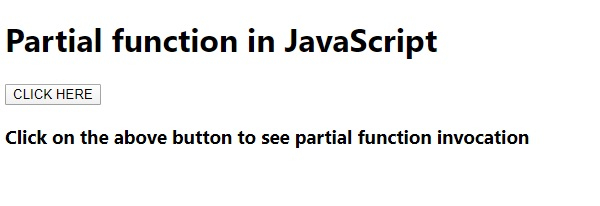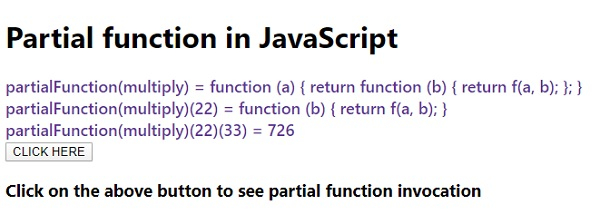# What are Partial functions in JavaScript?

JavascriptWeb DevelopmentObject Oriented Programming

Partial functions allow takes a function as an argument and along with it takes arguments of other types too. It then uses some of the arguments passed and returns a function that will take the remaining arguments. The function returned when invoked will call the parent function with the original and its own set of arguments.

Following is the code for partial functions in JavaScript −

## Example

Live Demo

<!DOCTYPE html>
<html lang="en">
<meta charset="UTF-8" />
<meta name="viewport" content="width=device-width, initial-scale=1.0" />
<title>Document</title>
<style>
body {
font-family: "Segoe UI", Tahoma, Geneva, Verdana, sans-serif;
}
.result {
font-size: 18px;
font-weight: 500;
color: rebeccapurple;
}
</style>
<body>
<h1>Partial function in JavaScript</h1>
<div class="result"></div>
<h3>
Click on the above button to see partial function invocation
</h3>
<script>
let resultEle = document.querySelector(".result");
function partialFunction(f) {
return function (a) {
return function (b) {
return f(a, b);
};
};
}
function multiply(a, b) {
return a * b;
}
resultEle.innerHTML = "partialFunction(multiply) = " + partialFunction(multiply) + "<br>";
resultEle.innerHTML += "partialFunction(multiply)(22) = " + partialFunction(multiply)(22) +
"<br>";
resultEle.innerHTML += "partialFunction(multiply)(22)(33) = " + partialFunction(multiply)(22)(33) + "<br>";
});
</script>
</body>
</html>

## Output

The above code will produce the following output −